# Difference between revisions of "Metrizable implies monotonically normal"

This article gives the statement and possibly, proof, of an implication relation between two topological space properties. That is, it states that every topological space satisfying the first topological space property must also satisfy the second topological space property
View all topological space property implications | View all topological space property non-implications
|
Property "Page" (as page type) with input value "{{{stronger}}}" contains invalid characters or is incomplete and therefore can cause unexpected results during a query or annotation process.
Property "Page" (as page type) with input value "{{{weaker}}}" contains invalid characters or is incomplete and therefore can cause unexpected results during a query or annotation process.

## Statement

Any metrizable space is monotonically normal. In fact, we can construct an explicit monotone normality operator using the metric.

## Definitions used

### Metrizable space

Further information: Metrizable space

### Monotonically normal space

Further information: Monotonically normal space

## Proof

### Construction of the operator

Suppose$(X,d)$ is a metric space. We construct a monotone normality operator on$X$ as follows. For any two closed subsets$A$ and$B$ of$X$:

• For every$a \in A$, let$U_a$ be the open ball about$a$ of radius$d(a,B)/2$. Here,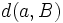$d(a,B)$ is the infimum of the distances$d(a,b)$ for$b \in B$. Note that since$B$ is closed and$a \notin B$,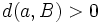$d(a,B) > 0$.
• Analogously, for every$b \in B$, define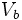$V_b$ to be the open ball about$b$ of radius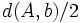$d(A,b)/2$.

Then, the sets: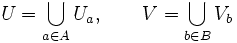$U = \bigcup_{a \in A} U_a, \qquad V = \bigcup_{b \in B} V_b$

are the required disjoint open subsets.

### Proof of disjointness

The disjointness of$U$ and$V$ follows from the triangle inequality. It suffices to prove that for every$a \in A$ and$b \in B$,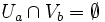$U_a \cap V_b = \emptyset$. To see this, note: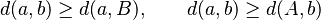$d(a,b) \ge d(a,B), \qquad d(a,b) \ge d(A,b)$

Thus:$d(a,b) \ge d(a,B)/2 + d(A,b)/2$

This, along with the triangle inequality yields that the balls$U_a$ and$V_b$ cannot intersect.

### Proof of monotonicity

We need to show that if$A' \subseteq A$ and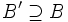$B' \supseteq B$, then the open set about$A$ becomes smaller, and the open set about$B$ becomes larger. This is clear from the definitions, because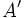$A'$ has fewer balls, and the radii of the balls also become smaller. Similarly$B'$ has more balls, and the radii of the balls also become larger.